Home Practice
For learners and parents For teachers and schools
Textbooks
Full catalogue
Pricing SupportLog in

We think you are located in United States. Is this correct?

# 8.2 Circle geometry

## 8.2 Circle geometry (EMBJ9)

Terminology

The following terms are regularly used when referring to circles:

• Arc — a portion of the circumference of a circle.
• Chord — a straight line joining the ends of an arc.
• Circumference — the perimeter or boundary line of a circle.
• Radius ($$r$$) — any straight line from the centre of the circle to a point on the circumference.
• Diameter — a special chord that passes through the centre of the circle. A diameter is a straight line segment from one point on the circumference to another point on the circumference that passes through the centre of the circle.
• Segment — part of the circle that is cut off by a chord. A chord divides a circle into two segments.
• Tangent — a straight line that makes contact with a circle at only one point on the circumference.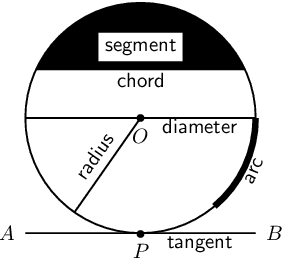temp text

Axioms

An axiom is an established or accepted principle. For this section, the following are accepted as axioms.

1. The theorem of Pythagoras states that the square of the hypotenuse of a right-angled triangle is equal to the sum of the squares of the other two sides.

$(AC)^2 = (AB)^2 + (BC)^2$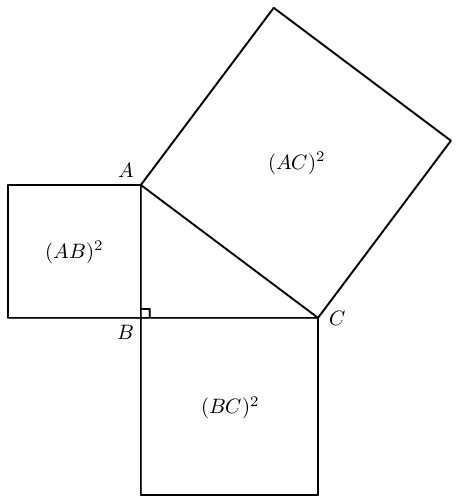2. A tangent is perpendicular to the radius ($$OT \perp ST$$), drawn at the point of contact with the circle.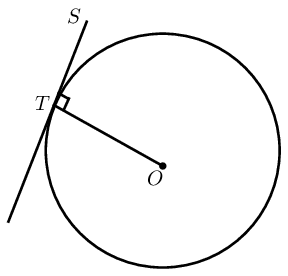### Theorems (EMBJB)

A theorem is a hypothesis (proposition) that can be shown to be true by accepted mathematical operations and arguments. A proof is the process of showing a theorem to be correct.

The converse of a theorem is the reverse of the hypothesis and the conclusion. For example, given the theorem “if $$A$$, then $$B$$”, the converse is “if $$B$$, then $$A$$”.

# Perpendicular line from circle centre bisects chord

If a line is drawn from the centre of a circle perpendicular to a chord, then it bisects the chord.

(Reason: $$\perp$$ from centre bisects chord)

Circle with centre $$O$$ and line $$OP$$ perpendicular to chord $$AB$$.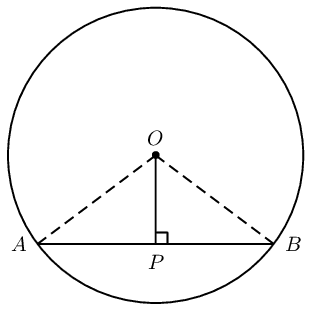$$AP = PB$$

Draw $$OA$$ and $$OB$$.

In $$\triangle OPA$$ and in $$\triangle OPB$$, $\begin{array}{rll} OA^2 &= OP^2 + AP^2 & \text{(Pythagoras)} \\ OB^2 &= OP^2 + BP^2 & \text{(Pythagoras)} \end{array}$ and $\begin{array}{rll} OA &= OB & \text{(equal radii)} \\ \therefore AP^2 &= BP^2 & \\ \therefore AP &= BP & \end{array}$ Therefore $$OP$$ bisects $$AB$$.

Alternative proof:

In $$\triangle OPA$$ and in $$\triangle OPB$$, $\begin{array}{rll} O\hat{P}A &= O\hat{P}B & (\text{given } OP \perp AB) \\ OA &= OB & \text{(equal radii)} \\ OP &= OP & \text{(common side)} \\ \therefore \triangle OPA & \equiv \triangle OPB & \text{(RHS)} \\ \therefore AP &= PB & \end{array}$ Therefore $$OP$$ bisects $$AB$$.

# (PROOF NOT FOR EXAMS) Converse: Line from circle centre to mid-point of chord is perpendicular

If a line is drawn from the centre of a circle to the mid-point of a chord, then the line is perpendicular to the chord.

(Reason: line from centre to mid-point $$\perp$$)

Circle with centre $$O$$ and line $$OP$$ to mid-point $$P$$ on chord $$AB$$.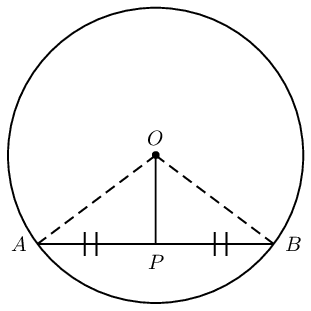$$OP \perp AB$$

Draw $$OA$$ and $$OB$$.

In $$\triangle OPA$$ and in $$\triangle OPB$$, $\begin{array}{rll} OA &= OB & \text{(equal radii)} \\ AP &= PB & \text{(given)} \\ OP &= OP & \text{(common side)} \\ \therefore \triangle OPA & \equiv \triangle OPB & \text{(SSS)} \\ \therefore O\hat{P}A &= O\hat{P}B & \\ \text{and } O\hat{P}A + O\hat{P}B &= \text{180}\text{°} & (\angle \text{ on str. line}) \\ \therefore O\hat{P}A = O\hat{P}B &= \text{90}\text{°} & \end{array}$ Therefore $$OP \perp AB$$.

# Theorem: Perpendicular bisector of chord passes through circle centre

If the perpendicular bisector of a chord is drawn, then the line will pass through the centre of the circle.

(Reason: $$\perp$$ bisector through centre)

Circle with mid-point $$P$$ on chord $$AB$$.

Line $$QP$$ is drawn such that $$Q\hat{P}A = Q\hat{P}B = \text{90}\text{°}$$.

Line $$RP$$ is drawn such that $$R\hat{P}A = R\hat{P}B = \text{90}\text{°}$$.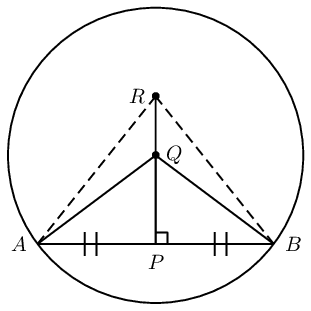Circle centre $$O$$ lies on the line $$PR$$

Draw lines $$QA$$ and $$QB$$.

Draw lines $$RA$$ and $$RB$$.

In $$\triangle QPA$$ and in $$\triangle QPB$$, $\begin{array}{rll} AP &= PB & \text{(given)} \\ QP &= QP & \text{(common side)} \\ Q\hat{P}A = Q\hat{P}B &= \text{90}\text{°} & \text{(given)} \\ \therefore \triangle QPA & \equiv \triangle QPB & \text{(SAS)} \\ \therefore QA &= QB & \end{array}$

Similarly it can be shown that in $$\triangle RPA$$ and in $$\triangle RPB$$, $$RA = RB$$.

We conclude that all the points that are equidistant from $$A$$ and $$B$$ will lie on the line $$PR$$ extended. Therefore the centre $$O$$, which is equidistant to all points on the circumference, must also lie on the line $$PR$$.

## Worked example 1: Perpendicular line from circle centre bisects chord

Given $$OQ \perp PR$$ and $$PR = 8$$ units, determine the value of $$x$$.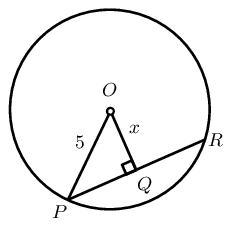### Use theorems and the given information to find all equal angles and sides on the diagram

$$PQ = QR = 4 \qquad (\perp \text{ from centre bisects chord})$$

### Solve for $$x$$

In $$\triangle OQP$$: $\begin{array}{rll} PQ &= 4 & (\perp \text{ from centre bisects chord}) \\ OP^2 &= OQ^2 + QP^2 & (\text{Pythagoras}) \\ 5^2 &= x^2 + 4^2 & \\ \therefore x^2 &= 25 - 16 & \\ x^2 &= 9 & \\ x &= 3 & \end{array}$

$$x = 3$$ units.

## Perpendicular line from center bisects chord

Textbook Exercise 8.1

In the circle with centre $$O$$, $$OQ \perp PR$$, $$OQ = 4$$ units and $$PR=10$$. Determine $$x$$.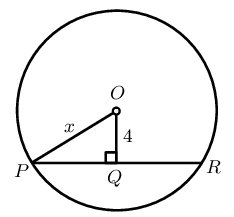$\begin{array}{rll} PR &= 10 & (\perp \text{ given }) \\ \therefore PQ &= 5 & (\perp \text{ from centre bisects chord}) \\ OP^2 &= OQ^2 + QP^2 & (\text{Pythagoras}) \\ x^2 &= 4^2 + 5^2 & \\ \therefore x^2 &= 25 + 16 & \\ x^2 &= 41 & \\ x &= \sqrt{41} & \end{array}$

In the circle with centre $$O$$ and radius $$= 10$$ units, $$OQ \perp PR$$ and $$PR=8$$. Determine $$x$$.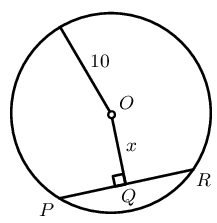$\begin{array}{rll} PR &= 8 & (\perp \text{ given }) \\ \therefore PQ &= 4 & (\perp \text{ from centre bisects chord}) \\ OP^2 &= OQ^2 + QP^2 & (\text{Pythagoras}) \\ 10^2 &= x^2 + 4^2 & \\ \therefore x^2 &= 100 - 16 & \\ x^2 &= 84 & \\ x &= \sqrt{84} & \end{array}$

In the circle with centre $$O$$, $$OQ \perp PR$$, $$PR = 12$$ units and $$SQ = 2$$ units. Determine $$x$$.$\begin{array}{rll} SO &= x -2 & \\ OP^2 &= OS^2 + SP^2 & (\text{Pythagoras}) \\ x^2 &= (x-2)^2 + 6^2 & \\ x^2 &= x^2 - 4x + 4 + 6^2 & \\ 4x &= 40 & \\ \therefore x &= 10 & \end{array}$

In the circle with centre $$O$$, $$OT \perp SQ$$, $$OT \perp PR$$, $$OP = 10$$ units, $$ST = 5$$ units and $$PU = 8$$ units. Determine $$TU$$.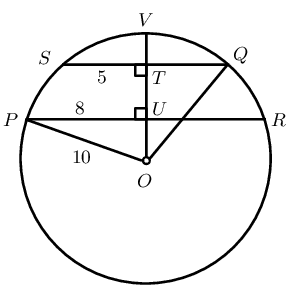$\begin{array}{rll} \text{In } \triangle POU, \quad OP^2 &= OU^2 + PU^2 & (\text{Pythagoras}) \\ 10^2 &= OU^2 + 8^2 & \\ 100 - 64 &= OU^2 & \\ \therefore OU^2 &= 36 & \\ \therefore OU &= 6 & \\ \text{In } \triangle QTO, \quad QO^2 &= OT^2 + TQ^2 & (\text{Pythagoras}) \\ 10^2 &= OT^2 + 5^2 & \\ 100 - 25 &= OT^2 & \\ \therefore OT^2 &= 75 & \\ \therefore OT &= \sqrt{75} & \\ \therefore TU &= OT - OU & \\ &= \sqrt{75} - 6 & \\ &= \text{2,66} & \end{array}$

In the circle with centre $$O$$, $$OT \perp QP$$, $$OS \perp PR$$, $$OT = 5$$ units, $$PQ = 24$$ units and $$PR = 25$$ units. Determine $$OS = x$$.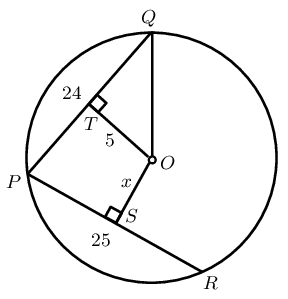$\begin{array}{rll} \text{In } \triangle QTO, \quad QO^2 &= OT^2 + QT^2 & (\text{Pythagoras}) \\ QO^2 &= 5^2 + 12^2 & \\ &= 25 + 144 & \\ \therefore QO^2 &= 169 & \\ \therefore QO &= 13 & \\ \text{In } \triangle OSR, \quad OR^2 &= SR^2 + OS^2 & (\text{Pythagoras}) \\ 13^2 &= \text{12,5}^2 + OS^2 & \\ \therefore OS^2 &= \text{12,75} & \\ \therefore OS &= \text{3,6} & \end{array}$

## Angles subtended by an arc at the centre and the circumference of a circle

1. Measure angles $$x$$ and $$y$$ in each of the following graphs: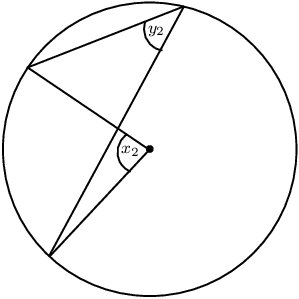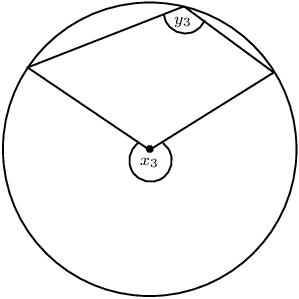2. Complete the table:

 $$x$$ $$y$$
3. Use your results to make a conjecture about the relationship between angles subtended by an arc at the centre of a circle and angles at the circumference of a circle.
4. Now draw three of your own similar diagrams and measure the angles to check your conjecture.

# Theorem: Angle at the centre of a circle is twice the size of the angle at the circumference

If an arc subtends an angle at the centre of a circle and at the circumference, then the angle at the centre is twice the size of the angle at the circumference.

(Reason: $$\angle \text{ at centre } = 2 \angle \text{ at circum.}$$)

Circle with centre $$O$$, arc $$AB$$ subtending $$A\hat{O}B$$ at the centre of the circle, and $$A\hat{P}B$$ at the circumference.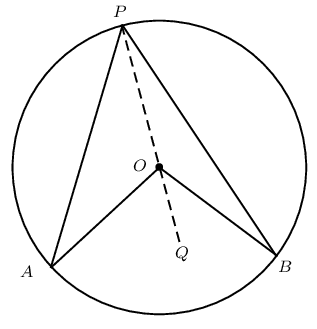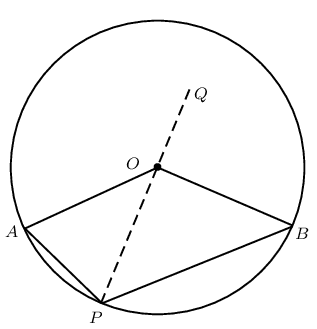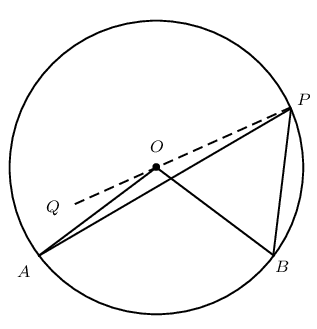$$A\hat{O}B= 2A\hat{P}B$$

Draw $$PO$$ extended to $$Q$$ and let $$A\hat{O}Q = \hat{O}_1$$ and $$B\hat{O}Q = \hat{O}_2$$.

$\begin{array}{rll} \hat{O}_1&= A\hat{P}O + P\hat{A}O & (\text{ext. } \angle \triangle = \text{sum int. opp. } \angle \text{s} ) \\ \text{and } A\hat{P}O &= P\hat{A}O & (\text{equal radii, isosceles } \triangle APO)\\ \therefore \hat{O}_1&= A\hat{P}O + A\hat{P}O & \\ \hat{O}_1&= 2A\hat{P}O & \end{array}$

Similarly, we can also show that $$\hat{O}_2 = 2B\hat{P}O$$.

For the first two diagrams shown above we have that: $\begin{array}{rll} A\hat{O}B &= \hat{O}_1 + \hat{O}_2 & \\ &= 2A\hat{P}O + 2B\hat{P}O & \\ &= 2(A\hat{P}O + B\hat{P}O) & \\ \therefore A\hat{O}B &= 2(A\hat{P}B) & \end{array}$ And for the last diagram: $\begin{array}{rll} A\hat{O}B &= \hat{O}_2 - \hat{O}_1 & \\ &= 2B\hat{P}O - 2A\hat{P}O & \\ &= 2(B\hat{P}O - A\hat{P}O) & \\ \therefore A\hat{O}B &= 2(A\hat{P}B) & \end{array}$

## Worked example 2: Angle at the centre of circle is twice angle at circumference

Given $$HK$$, the diameter of the circle passing through centre $$O$$.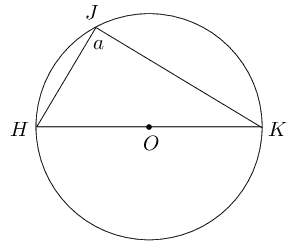### Solve for $$a$$

In $$\triangle HJK$$: $\begin{array}{rll} H\hat{O}K &= \text{180}\text{°} & (\angle \text{ on str. line)} \\ &= 2a & (\angle \text{ at centre } = 2 \angle \text{ at circum.}) \\ \therefore 2a &= \text{180}\text{°} & \\ a &= \frac{\text{180}\text{°}}{2} & \\ &= \text{90}\text{°} & \end{array}$

### Conclusion

The diameter of a circle subtends a right angle at the circumference (angles in a semi-circle).

## Angle at the centre of circle is twice angle at circumference

Textbook Exercise 8.2

Given $$O$$ is the centre of the circle, determine the unknown angle in each of the following diagrams: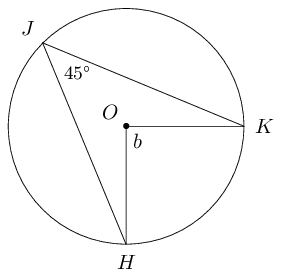$\begin{array}{rll} b &= 2 \times \text{45}\text{°} & (\angle \text{ at centre } = 2 \angle \text{ at circum.}) \\ \therefore b &= \text{90}\text{°} & \end{array}$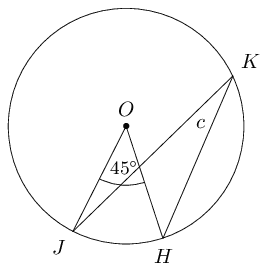$\begin{array}{rll} c &= \frac{1}{2} \times \text{45}\text{°} & (\angle \text{ at centre } = 2 \angle \text{ at circum.}) \\ \therefore c &= \text{22,5}\text{°} & \end{array}$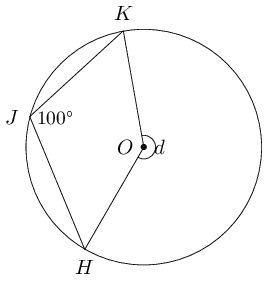$\begin{array}{rll} d &= 2 \times \text{100}\text{°} & (\angle \text{ at centre } = 2 \angle \text{ at circum.}) \\ \therefore d &= \text{200}\text{°} & \end{array}$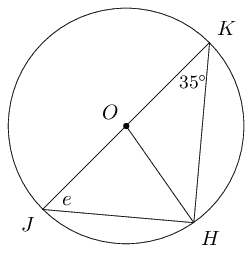$\begin{array}{rll} e &= \text{100}\text{°} - \text{90}\text{°} - \text{35}\text{°} & (\angle \text{ in semi circle} ) \\ \therefore e &= \text{55}\text{°} & \end{array}$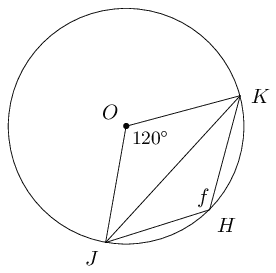$\begin{array}{rll} f &= \frac{1}{2} \times \text{240}\text{°} & (\angle \text{ at centre } = 2 \angle \text{ at circum.}) \\ \therefore f &= \text{120}\text{°} & \end{array}$

## Subtended angles in the same segment of a circle

1. Measure angles $$a$$, $$b$$, $$c$$, $$d$$ and $$e$$ in the diagram below:2. Choose any two points on the circumference of the circle and label them $$A$$ and $$B$$.

3. Draw $$AP$$ and $$BP$$, and measure $$A\hat{P}B$$.

4. Draw $$AQ$$ and $$BQ$$, and measure $$A\hat{Q}B$$.

5. What do you observe? Make a conjecture about these types of angles.

# Theorem: Subtended angles in the same segment of a circle are equal

If the angles subtended by a chord of the circle are on the same side of the chord, then the angles are equal.

(Reason: $$\angle$$s in same seg.)

Circle with centre $$O$$, and points $$P$$ and $$Q$$ on the circumference of the circle. Arc $$AB$$ subtends $$A\hat{P}B$$ and $$A\hat{Q}B$$ in the same segment of the circle.$$A\hat{P}B = A\hat{Q}B$$
$\begin{array}{rll} A\hat{O}B &= 2 A\hat{P}B & (\angle \text{ at centre } = 2 \angle \text{ at circum.}) \\ A\hat{O}B &= 2 A\hat{Q}B & (\angle \text{ at centre } = 2 \angle \text{ at circum.}) \\ \therefore 2 A\hat{P}B &= 2 A\hat{Q}B & \\ A\hat{P}B &= A\hat{Q}B & \end{array}$

Equal arcs subtend equal angles

From the theorem above we can deduce that if angles at the circumference of a circle are subtended by arcs of equal length, then the angles are equal. In the figure below, notice that if we were to move the two chords with equal length closer to each other, until they overlap, we would have the same situation as with the theorem above. This shows that the angles subtended by arcs of equal length are also equal.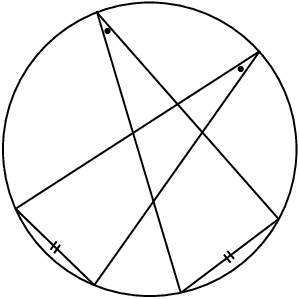# (PROOF NOT FOR EXAMS) Converse: Concyclic points

If a line segment subtends equal angles at two other points on the same side of the line segment, then these four points are concyclic (lie on a circle).

Line segment $$AB$$ subtending equal angles at points $$P$$ and $$Q$$ on the same side of the line segment $$AB$$.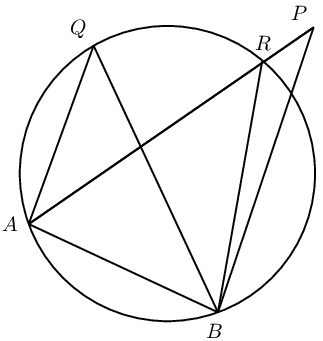$$A$$, $$B$$, $$P$$ and $$Q$$ lie on a circle.

Points on the circumference of a circle: we know that there are only two possible options regarding a given point — it either lies on circumference or it does not.

We will assume that point $$P$$ does not lie on the circumference.

We draw a circle that cuts $$AP$$ at $$R$$ and passes through $$A$$, $$B$$ and $$Q$$. $\begin{array}{rll} A\hat{Q}B &= A\hat{R}B & (\angle \text{s in same seg.}) \\ \text{but } A\hat{Q}B &= A\hat{P}B & (\text{given}) \\ \therefore A\hat{R}B &= A\hat{P}B & \\ \text{but } A\hat{R}B &= A\hat{P}B + R\hat{B}P & (\text{ext. } \angle \triangle = \text{sum int. opp.}) \\ \therefore R\hat{B}P &= \text{0}\text{°} & \end{array}$ Therefore the assumption that the circle does not pass through $$P$$ must be false.

We can conclude that $$A$$, $$B$$, $$Q$$ and $$P$$ lie on a circle ($$A$$, $$B$$, $$Q$$ and $$P$$ are concyclic).

## Worked example 3: Concyclic points

Given $$FH \parallel EI$$ and $$E\hat{I}F = \text{15}\text{°}$$, determine the value of $$b$$.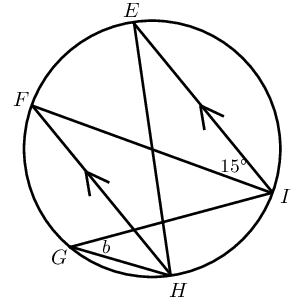### Solve for $$b$$

$\begin{array}{rll} H\hat{F}I &= \text{15}\text{°} & (\text{alt. } \angle, FH \parallel EI) \\ \text{and } b &= H\hat{F}I & (\angle \text{s in same seg.}) \\ \therefore b &= \text{15}\text{°} & \end{array}$

## Subtended angles in the same segment

Textbook Exercise 8.3

Find the values of the unknown angles.$\begin{array}{rll} a &= \text{21}\text{°} & (\angle \text{s in same seg.}) \end{array}$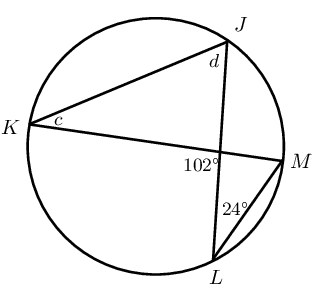$\begin{array}{rll} c &= \text{24}\text{°} & (\angle \text{s in same seg.}) \\ d &= \text{102}\text{°} - \text{24}\text{°} & (\text{ext. } \angle \triangle = \text{sum int. opp.}) \\ \therefore d &= \text{78}\text{°} & \\ \end{array}$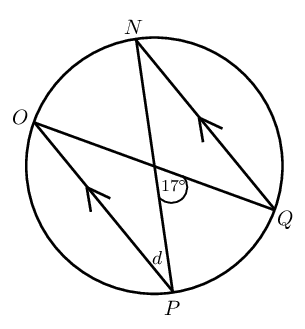$\begin{array}{rll} d &= \hat{N} & (\text{alt. } \angle, PO \parallel QN ) \\ \hat{N} &= \frac{1}{2} \times \text{17}\text{°} & (\angle \text{ at centre } = 2 \angle \text{ at circum.}) \\ \hat{O} &= \hat{N} & (\angle \text{s in same seg.}) \\ \text{17}\text{°} &= \hat{O} + d & ( \text{ ext. angle of } \triangle) \\ \therefore 2d &= \text{17}\text{°} & \\ \therefore d &= \text{8,5}\text{°} & (\text{alt. } \angle, PO \parallel QN ) \end{array}$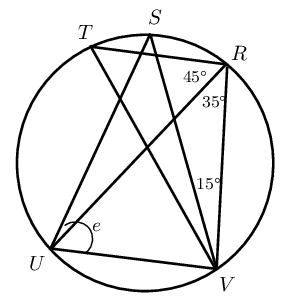Given $$T\hat{V}S = S\hat{V}R$$, determine the value of $$e$$.

$\begin{array}{rll} \text{In } \triangle TRV, \hat{T} &= \text{180}\text{°} - (\text{80}\text{°} + \text{30}\text{°}) & (\angle \text{s sum of } \triangle) \\ \therefore \hat{T} &= \text{70}\text{°} & \\ \therefore e &= \text{15}\text{°} + \text{70}\text{°} & \\ &= \text{85}\text{°} & \\ \end{array}$

Is $$TV$$ a diameter of the circle? Explain your answer.

No, since $$\text{45}\text{°} + \text{35}\text{°} \ne \text{90}\text{°}$$

Given circle with centre $$O$$, $$WT = TY$$ and $$X\hat{W}T = \text{35}\text{°}$$. Determine $$f$$.$\begin{array}{rll} \text{In } \triangle WTZ &\text{ and in } \triangle YTZ, \\ WT &= YT & (\text{ given }) \\ ZT &= ZT & (\text{ common side }) \\ Y\hat{T}Z = W\hat{T}Z &= \text{90}\text{°} & (\text{ line from circle centre to mid-point }) \\ \therefore T\hat{Z}Y &= T\hat{Z}W & (\text{ SAS }) \\ T\hat{Z}Y = T\hat{Z}W &= f & \\ \text{And } T\hat{Z}Y &= \text{35}\text{°} & (\angle \text{s in same seg.}) \\ \therefore T\hat{Z}W = f &= \text{35}\text{°} & \end{array}$

Cyclic quadrilaterals are quadrilaterals with all four vertices lying on the circumference of a circle (concyclic).

Consider the diagrams given below:

 Circle $$\text{1}$$ Circle $$\text{2}$$ Circle $$\text{3}$$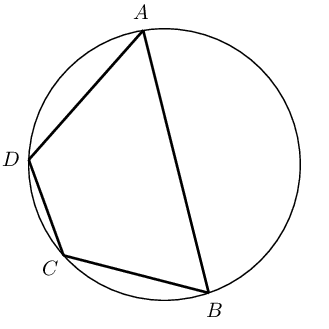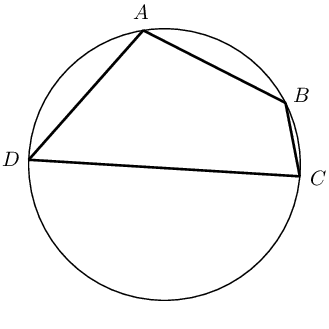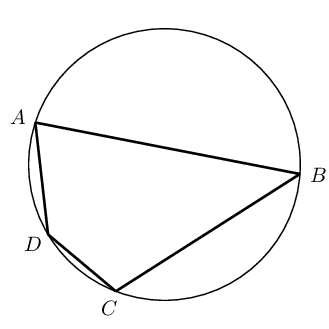1. Complete the following:

$$ABCD$$ is a cyclic quadrilateral because $$\ldots \ldots$$

2. Complete the table:

 Circle $$\text{1}$$ Circle $$\text{2}$$ Circle $$\text{3}$$ $$\hat{A} =$$ $$\hat{B} =$$ $$\hat{C} =$$ $$\hat{D} =$$ $$\hat{A} + \hat{C} =$$ $$\hat{B} + \hat{D} =$$

# Theorem: Opposite angles of a cyclic quadrilateral

The opposite angles of a cyclic quadrilateral are supplementary.

(Reason: opp. $$\angle$$s cyclic quad.)

Circle with centre $$O$$ with points $$A, B, P$$ and $$Q$$ on the circumference such that $$ABPQ$$ is a cyclic quadrilateral.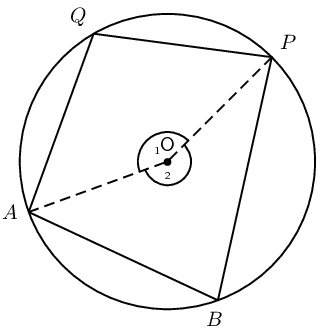$$A\hat{B}P + A\hat{Q}P = \text{180}\text{°}$$ and $$Q\hat{A}B + Q\hat{P}B = \text{180}\text{°}$$

Draw $$AO$$ and $$OP$$. Label $$\hat{O}_1$$ and $$\hat{O}_2$$. $\begin{array}{rll} \hat{O}_1 &= 2A\hat{B}P & (\angle\text{ at centre} = 2\angle \text{ at circum.})\\ \hat{O}_2 &= 2A\hat{Q}P & (\angle\text{ at centre} = 2\angle \text{ at circum.})\\ \text{and } \hat{O}_1 + \hat{O}_2 &= \text{360}\text{°} & (\angle\text{s around a point}) \\ \therefore 2A\hat{B}P + 2A\hat{Q}P &= \text{360}\text{°} & \\ A\hat{B}P + A\hat{Q}P &= \text{180}\text{°} & \end{array}$ Similarly, we can show that $$Q\hat{A}B + Q\hat{P}B = \text{180}\text{°}$$.

Converse: interior opposite angles of a quadrilateral

If the interior opposite angles of a quadrilateral are supplementary, then the quadrilateral is cyclic.

Exterior angle of a cyclic quadrilateral

If a quadrilateral is cyclic, then the exterior angle is equal to the interior opposite angle.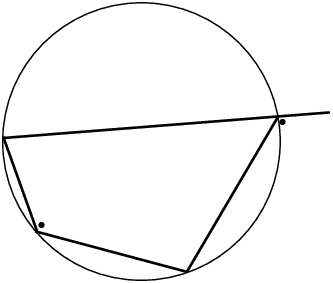## Worked example 4: Opposite angles of a cyclic quadrilateral

Given the circle with centre $$O$$ and cyclic quadrilateral $$PQRS$$. $$SQ$$ is drawn and $$S\hat{P}Q = \text{34}\text{°}$$. Determine the values of $$a$$, $$b$$ and $$c$$.### Solve for $$b$$

$\begin{array}{rll} S\hat{P}Q + c &= \text{180}\text{°} & (\text{opp. } \angle \text{s cyclic quad supp.}) \\ \therefore c &= \text{180}\text{°} - \text{34}\text{°} & \\ &= \text{146}\text{°} & \end{array}$ $\begin{array}{rll} a &= \text{90}\text{°} & (\angle \text{ in semi circle}) \end{array}$ In $$\triangle PSQ$$: $\begin{array}{rll} a + b + \text{34}\text{°} &= \text{180}\text{°} & (\angle \text{ sum of } \triangle) \\ \therefore b &= \text{180}\text{°} - \text{90}\text{°} - \text{34}\text{°} & \\ &= \text{56}\text{°} & \end{array}$

Methods for proving a quadrilateral is cyclic

There are three ways to prove that a quadrilateral is a cyclic quadrilateral:

 Method of proof ReasonIf $$\hat{P} + \hat{R} = \text{180}\text{°}$$ or $$\hat{S} + \hat{Q} = \text{180}\text{°}$$, then $$PQRS$$ is a cyclic quad. opp. int. angles suppl.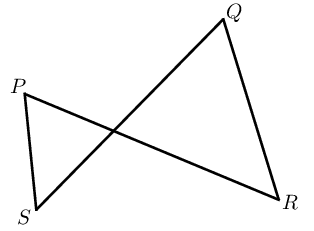If $$\hat{P} = \hat{Q}$$ or $$\hat{S} = \hat{R}$$, then $$PQRS$$ is a cyclic quad. angles in the same seg.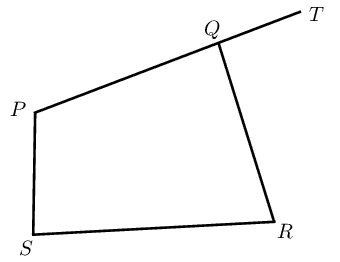If $$T\hat{Q}R = \hat{S}$$, then $$PQRS$$ is a cyclic quad. ext. angle equal to int. opp. angle

Prove that $$ABDE$$ is a cyclic quadrilateral.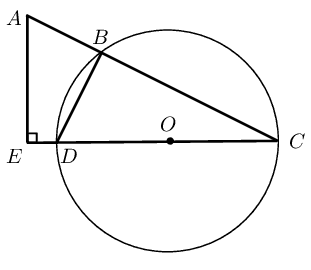### Prove that $$ABDE$$ is a cyclic quadrilateral

$\begin{array}{rll} D\hat{B}C &= \text{90}\text{°} & (\angle \text{ in semi circle}) \\ \text{and } \hat{E} &= \text{90}\text{°} & (\text{given}) \\ \therefore D\hat{B}C &= \hat{E} & \\ \therefore ABDE \text{ is a cyclic} & \text{quadrilateral} & \text{(ext.\@ $$\angle$$ equals int.\@ opp.\@ $$\angle$$)} \end{array}$

Textbook Exercise 8.4

Find the values of the unknown angles.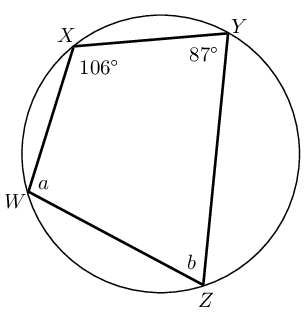$\begin{array}{rll} a + \text{87}\text{°} &= \text{180}\text{°} & (\text{opp. angles of cyclic quad. supp. }) \\ \therefore a &= \text{93}\text{°} & \\ b + \text{106}\text{°} &= \text{180}\text{°} & (\text{opp. angles of cyclic quad. supp. }) \\ \therefore b &= \text{74}\text{°} & \end{array}$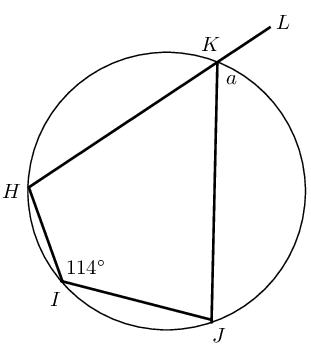$\begin{array}{rll} a &= H\hat{I}J & \angle (\text{ ext. angle cyclic quad = int. opp }) \\ &= \text{114}\text{°} & \end{array}$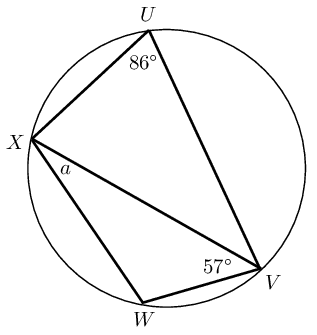$\begin{array}{rll} \hat{W} + \text{86}\text{°} &= \text{180}\text{°} & (\text{opp. angles of cyclic quad. supp. }) \\ \therefore \hat{W} &= \text{94}\text{°} & \\ a + \hat{W} + \text{57}\text{°} &= \text{180}\text{°} & (\text{angles sum of } \triangle) \\ \therefore a &= \text{29}\text{°} & \end{array}$

Prove that $$ABCD$$ is a cyclic quadrilateral: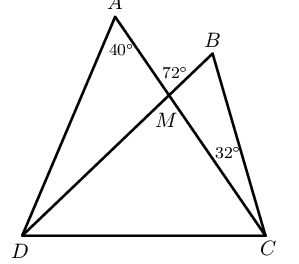$\begin{array}{rll} A\hat{M}B &= \text{32}\text{°} + D\hat{B}C & (\text{ext angle of } \triangle = \text{sum int. opp. angles}) \\ \therefore \text{72}\text{°} &= \text{32}\text{°} + D\hat{B}C & \\ \therefore D\hat{B}C &= \text{40}\text{°} & (\text{angles sum of } \triangle) \\ \therefore D\hat{B}C &= D\hat{A}C & \\ \text{Therefore } ABCD &= \text{ is cyclic quad. } & ( \text{ angles in same seg.}) \end{array}$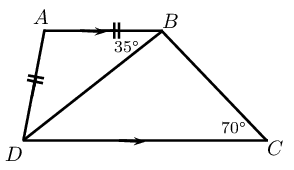$\begin{array}{rll} \text{In } \triangle ABD, \quad A\hat{B}D = A\hat{D}B &= \text{35}\text{°} & (\angle \text{s opp. equal sides }) \\ \therefore \text{35}\text{°} + \text{35}\text{°} + D\hat{A}B &= \text{180}\text{°} & (\angle \text{s sum of } \triangle) \\ \therefore D\hat{A}B &= \text{110}\text{°} & (\text{angles sum of } \triangle) \\ \text{And } D\hat{A}B + D\hat{C}B &= \text{180}\text{°} & \\ \text{Therefore } ABCD &= \text{ is cyclic quad. } & ( \text{ opp. int. angles supp.}) \end{array}$

Tangent line to a circle

A tangent is a line that touches the circumference of a circle at only one place. The radius of a circle is perpendicular to the tangent at the point of contact.# Theorem: Two tangents drawn from the same point outside a circle

If two tangents are drawn from the same point outside a circle, then they are equal in length.

(Reason: tangents from same point equal)

Circle with centre $$O$$ and tangents $$PA$$ and $$PB$$, where $$A$$ and $$B$$ are the respective points of contact for the two lines.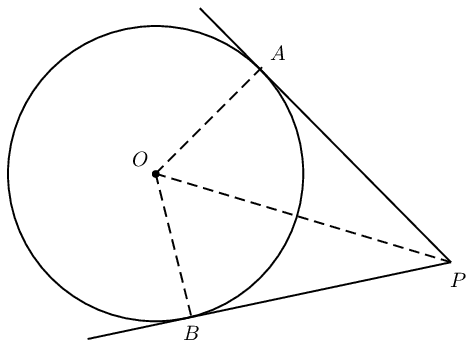$$AP = BP$$

In $$\triangle AOP$$ and $$\triangle BOP$$, $\begin{array}{rll} O\hat{A}P = O\hat{B}P &= \text{90}\text{°} & (\text{tangent} \perp \text{radius})\\ AO &= BO & (\text{equal radii}) \\ OP &= OP & (\text{common side}) \\ \therefore \triangle AOP &\equiv \triangle BOP & (\text{RHS}) \\ \therefore AP &= BP & \end{array}$

## Worked example 6: Tangents from the same point outside a circle

In the diagram below $$AE = \text{5}\text{ cm}$$, $$AC = \text{8}\text{ cm}$$ and $$CE = \text{9}\text{ cm}$$. Determine the values of $$a$$, $$b$$ and $$c$$.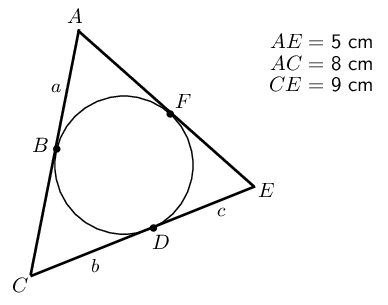### Solve for $$a$$, $$b$$ and $$c$$

$\begin{array}{rll} AB = AF&= a & (\text{tangents from } A) \\ EF = ED&= c & (\text{tangents from } E) \\ CB = CD&= b & (\text{tangents from } C) \\ \therefore AE = a + c &= 5 & \\ \text{and } AC = a + b &= 8 & \\ \text{and } CE = b + c &= 9 & \end{array}$

### Solve for the unknown variables using simultaneous equations

$\begin{array}{rll} a + c &= 5 & \ldots (1)\\ a + b &= 8 & \ldots (2)\\ b + c &= 9 & \ldots (3) \end{array}$

Subtract equation $$(1)$$ from equation $$(2)$$ and then substitute into equation $$(3)$$:

$\begin{array}{rll} (2) - (1) \quad b-c &= 8-5 & \\ &= 3 & \\ \therefore b &= c + 3 & \\ \text{Substitute into } (3) \quad c + 3 + c &= 9 & \\ 2c &= 6 & \\ c &= 3 & \\ \therefore a &= 2 & \\ \text{ and } b &= 6 & \end{array}$

## Tangents to a circle

Textbook Exercise 8.5

Find the values of the unknown lengths.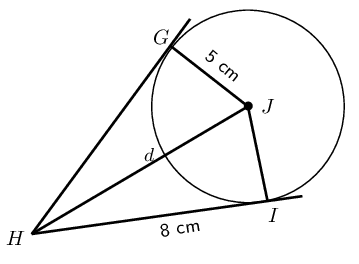$\begin{array}{rll} HI &= HG & (\text{tangents same pt. }) \\ \text{In } \triangle HIJ, \quad d^2 &= 8^2 + 5^2 & (\text{Pythagoras, radius perp. tangent }) \\ \therefore d^2 &= 89 & \\ \therefore d &= \text{9,4}\text{ cm} & \end{array}$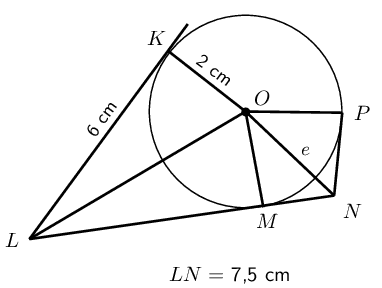$\begin{array}{rll} LM = LK &= 6 & (\text{tangents same pt. }) \\ LN &= \text{7,5}\text{ cm} & (\text{ given }) \\ \therefore MN = \text{7,5} - \text{6} &= \text{1,5}\text{ cm} & \\ OM &= \text{2}\text{ cm} & (\text{ radius }) \\ \text{In } \triangle OMN, \quad e^2 &= 2^2 + (\text{1,5})^2 & (\text{Pythagoras, radius perp. tangent }) \\ \therefore e^2 &= \text{6,25} & \\ \therefore e &= \text{2,5}\text{ cm} & \end{array}$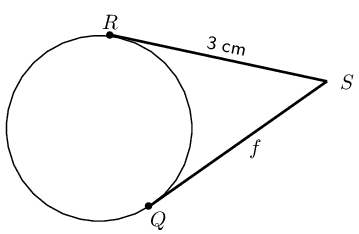$$f=\text{3}\text{ cm}$$

## Tangent-chord theorem

Consider the diagrams given below:

 Diagram $$\text{1}$$ Diagram $$\text{2}$$ Diagram $$\text{3}$$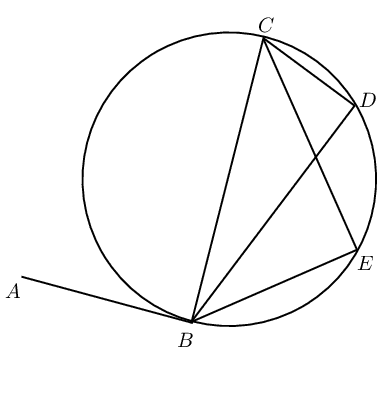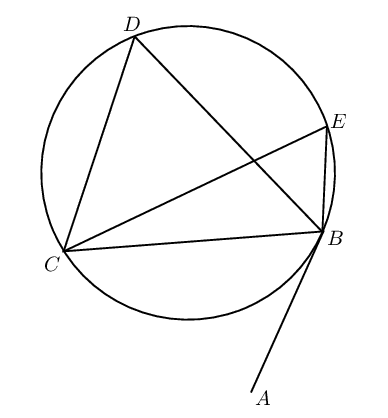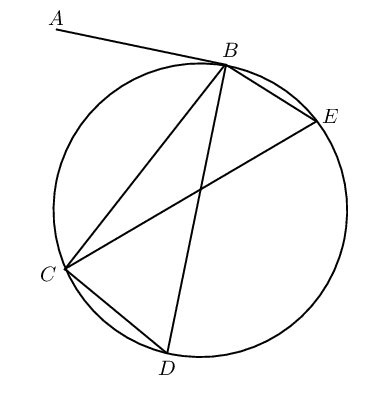1. Measure the following angles with a protractor and complete the table:

 Diagram $$\text{1}$$ Diagram $$\text{2}$$ Diagram $$\text{3}$$ $$A\hat{B}C =$$ $$\hat{D} =$$ $$\hat{E} =$$
2. Use your results to complete the following: the angle between a tangent to a circle and a chord is $$\ldots \ldots$$ to the angle in the alternate segment.

# Theorem: Tangent-chord theorem

The angle between a tangent to a circle and a chord drawn at the point of contact, is equal to the angle which the chord subtends in the alternate segment.

(Reason: tan. chord theorem)

Circle with centre $$O$$ and tangent $$SR$$ touching the circle at $$B$$. Chord $$AB$$ subtends $$\hat{P}_1$$ and $$\hat{Q}_1$$.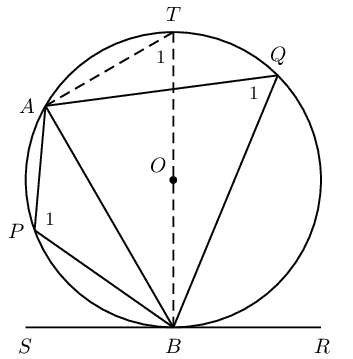1. $$A\hat{B}R = A\hat{P}B$$
2. $$A\hat{B}S = A\hat{Q}B$$

Draw diameter $$BT$$ and join $$T$$ to $$A$$.

Let $$A\hat{T}B = T_1$$. $\begin{array}{rll} A\hat{B}S + A\hat{B}T &= \text{90}\text{°} & (\text{tangent} \perp \text{radius}) \\ B\hat{A}T &= \text{90}\text{°} & (\angle \text{ in semi circle}) \\ \therefore A\hat{B}T + T_1 &= \text{90}\text{°} & (\angle \text{ sum of } \triangle BAT) \\ \therefore A\hat{B}S &= T_1 & \\ \text{but } Q_1 &= T_1 & (\angle \text{s in same segment}) \\ \therefore Q_1 &= A\hat{B}S & \end{array}$ $\begin{array}{rll} A\hat{B}S + A\hat{B}R &= \text{180}\text{°} & (\angle \text{s on str. line}) \\ \hat{Q}_1 + \hat{P}_1 &= \text{180}\text{°} & (\text{opp. } \angle \text{s cyclic quad. supp.}) \\ \therefore A\hat{B}S + A\hat{B}R &= Q_1 + P_1 & \\ \text{and } A\hat{B}S &= Q_1 & \\ \therefore A\hat{B}R &= P_1 & \end{array}$

## Worked example 7: Tangent-chord theorem

Determine the values of $$h$$ and $$s$$.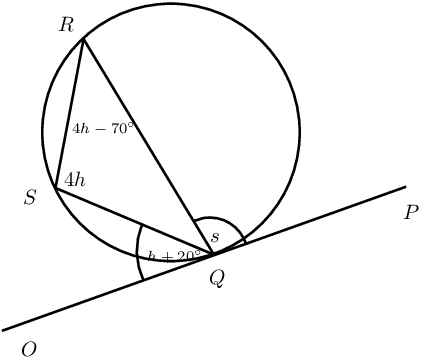### Solve for $$h$$

$\begin{array}{rll} O\hat{Q}S &= S\hat{R}Q & (\text{tangent chord theorem}) \\ h + \text{20}\text{°} &= 4h - \text{70}\text{°} & \\ \text{90}\text{°} &= 3h & \\ \therefore h &= \text{30}\text{°} & \end{array}$

### Solve for $$s$$

$\begin{array}{rll} P\hat{Q}R &= Q\hat{S}R & (\text{tangent chord theorem}) \\ s &= 4h & \\ &= 4(\text{30}\text{°}) & \\ &= \text{120}\text{°} & \end{array}$

## Tangent-chord theorem

Textbook Exercise 8.6

Find the values of the unknown letters, stating reasons.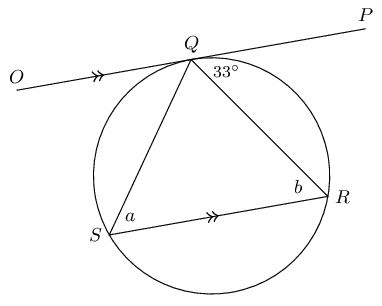$\begin{array}{rll} a &= \text{33}\text{°} & (\text{tangent-chord }) \\ b &= \text{33}\text{°} & (\text{alt. angles, } OP \parallel SR ) \end{array}$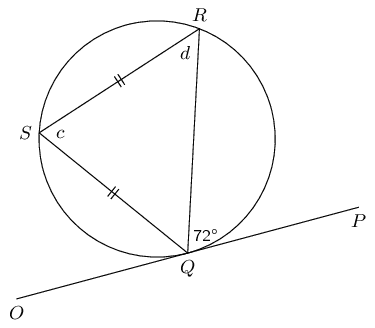$\begin{array}{rll} c &= \text{72}\text{°} & (\text{tangent-chord}) \\ d &= \dfrac{\text{180}\text{°} - \text{72}\text{°} }{2} & (\text{isosceles triangle}) \\ &= \text{54}\text{°} & (\text{alt. angles, } OP \parallel SR ) \end{array}$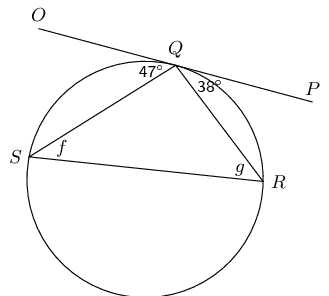$\begin{array}{rll} f &= \text{38}\text{°} & (\text{tangent-chord}) \\ g &= \text{47}\text{°} & (\text{tangent-chord}) \end{array}$$\begin{array}{rll} \hat{O}_1 = \hat{Q}_1 &= \text{66}\text{°} & (\text{isosceles, tangent-chord}) \\ \therefore l &= \text{180}\text{°} - 2 \times \text{66}\text{°} & (\text{angles sum } \triangle) \\ &= \text{48}\text{°} & \end{array}$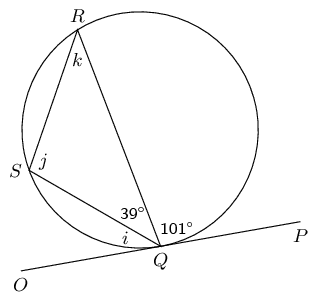$\begin{array}{rll} i &= \text{180}\text{°} - \text{101}\text{°} - \text{39}\text{°} & (\angle \text{s on str. line }) \\ \therefore i &= \text{40}\text{°} & \\ j &= \text{101}\text{°} & (\text{ tangent-chord}) \\ k = i &= \text{40}\text{°} & (\text{ tangent-chord}) \end{array}$$\begin{array}{rll} n &= \text{34}\text{°} & (\text{ tangent-chord}) \\ o &= \text{180}\text{°} - \text{90}\text{°} - \text{34}\text{°} & (\text{angles sum } \triangle) \\ \therefore o &= \text{56}\text{°} & \\ m &= \text{56}\text{°} & (\text{ tangent-chord}) \end{array}$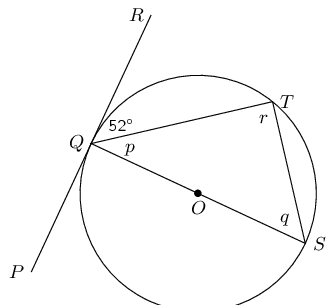$\begin{array}{rll} q &= \text{52}\text{°} & (\text{ tangent-chord}) \\ p &= \text{90}\text{°} - \text{52}\text{°} & (\text{tangent perp. radius }) \\ \therefore p &= \text{38}\text{°} & \\ r &= \text{90}\text{°} & ( \angle \text{ in semi-circle}) \end{array}$

$$O$$ is the centre of the circle and $$SPT$$ is a tangent, with $$OP \perp ST$$. Determine $$a$$, $$b$$ and $$c$$, giving reasons.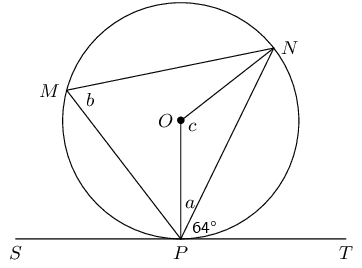$\begin{array}{rll} a &= \text{90}\text{°} - \text{64}\text{°} & (\text{tangent perp. radius}) \\ &= \text{26}\text{°} & \\ b &= \text{64}\text{°} & (\text{tangent chord}) \\ c &= 2 \times \text{64}\text{°} & (\angle\text{ at centre} = 2\angle \text{ at circum.}) \\ &= \text{128}\text{°} & \\ \end{array}$Given $$AB = AC$$, $$AP \parallel BC$$ and $$\hat{A}_2 = \hat{B}_2$$. Prove:

$$PAL$$ is a tangent to the circle $$ABC$$.

$\begin{array}{rll} \hat{A}_1 &= A\hat{C}B & (\text{alt. angles}, AP \parallel BC) \\ A\hat{C}B &= A\hat{B}C & (\angle \text{s opp. equal sides }, AB = AC) \\ \therefore \hat{A}_1 &= A\hat{B}C & \\ \text{Therefore } PAL & \text{is a tangent to circle } ABC & (\angle\text{ between line chord} = \angle\text{ in alt. seg.}) \end{array}$

$$AB$$ is a tangent to the circle $$ADP$$.

$\begin{array}{rll} \hat{A}_2 &= \hat{B}_2 & (\text{ given }) \\ \text{And } A\hat{P}B &= \hat{B}_2 & (\text{alt. angles}, AP \parallel BC) \\ \text{Therefore } A\hat{P}B &= \hat{A}_2 ABC & \\ \text{Therefore } AB & \text{is a tangent to circle } ADP & (\angle\text{ between line chord} = \angle\text{ in alt. seg.}) \end{array}$

Converse: tangent-chord theorem

If a line drawn through the end point of a chord forms an angle equal to the angle subtended by the chord in the alternate segment, then the line is a tangent to the circle.

(Reason: $$\angle$$ between line and chord $$= \angle$$ in alt. seg. )

## Worked example 8: Applying the theorems$$BD$$ is a tangent to the circle with centre $$O$$, with $$BO \perp AD$$.

Prove that:

1. $$CFOE$$ is a cyclic quadrilateral

2. $$FB=BC$$

3. $$\angle A\hat{O}C = 2 B\hat{F}C$$

4. Will $$DC$$ be a tangent to the circle passing through $$C,F,O$$ and $$E$$? Motivate your answer.

### Prove $$CFOE$$ is a cyclic quadrilateral by showing opposite angles are supplementary

$\begin{array}{rll} BO & \perp OD & (\text{given}) \\ \therefore F\hat{O}E &= \text{90}\text{°} & \\ F\hat{C}E &= \text{90}\text{°} & (\angle \text{ in semi circle}) \\ \therefore CFOE & \text{is a cyclic quad.} & (\text{opp. } \angle \text{s suppl.}) \end{array}$

### Prove $$BFC$$ is an isosceles triangle

To show that $$FB = BC$$ we first prove $$\triangle BFC$$ is an isosceles triangle by showing that $$B\hat{F}C = B\hat{C}F$$.

$\begin{array}{rll} B\hat{C}F &= C\hat{E}O & (\text{tangent-chord}) \\ C\hat{E}O &= B\hat{F}C & (\text{ext. } \angle \text{ cyclic quad. } CFOE) \\ \therefore B\hat{F}C &= B\hat{C}F \\ \therefore FB &= BC & (\triangle BFC \text{ isosceles}) \end{array}$

### Prove $$A\hat{O}C = 2 B\hat{F}C$$

$\begin{array}{rll} A\hat{O}C &= 2 A\hat{E}C & (\angle \text{ at centre } = 2 \angle \text{ at circum.}) \\ \text{and } A\hat{E}C &= B\hat{F}C & (\text{ext. } \angle \text{ cyclic quad. } CFOE) \\ \therefore A\hat{O}C &= 2 B\hat{F}C \end{array}$

### Determine if $$DC$$ is a tangent to the circle through $$C$$, $$F$$, $$O$$ and $$E$$

Let us assume that $$DC$$ is a tangent to the circle passing through the points $$C$$, $$F$$, $$O$$ and $$E$$: $\begin{array}{rll} \therefore D\hat{C}E = C\hat{O}E \quad (\text{tangent-chord}) \end{array}$ And using the circle with centre $$O$$ and tangent $$BD$$ we have that: $\begin{array}{rll} D\hat{C}E &= C\hat{A}E & (\text{tangent-chord}) \\ \text{but } C\hat{A}E &= \frac{1}{2} C\hat{O}E & (\angle \text{ at centre} = 2 \angle \text{ at circum.}) \\ \therefore D\hat{C}E &\ne C\hat{O}E & \end{array}$ Therefore our assumption is not correct and we can conclude that $$DC$$ is not a tangent to the circle passing through the points $$C$$, $$F$$, $$O$$ and $$E$$.

## Worked example 9: Applying the theorems$$FD$$ is drawn parallel to the tangent $$CB$$

Prove that:

1. $$FADE$$ is a cyclic quadrilateral

2. $$F\hat{E}A = \hat{B}$$

### Prove $$FADE$$ is a cyclic quadrilateral using angles in the same segment

$\begin{array}{rll} F\hat{D}C &= D\hat{C}B & (\text{alt. } \angle\text{s } FD \parallel CB) \\ \text{and } D\hat{C}B &= C\hat{A}E & (\text{tangent-chord}) \\ \therefore F\hat{D}C &= C\hat{A}E \\ \therefore FADE & \text{is a cyclic quad.} & (\angle \text{s in same seg.}) \end{array}$

### Prove $$F\hat{E}A = \hat{B}$$

$\begin{array}{rll} F\hat{D}A &= \hat{B} & (\text{corresp. } \angle\text{s } FD \parallel CB) \\ \text{and } F\hat{E}A &= F\hat{D}A & (\angle \text{s same seg.\@ cyclic quad.\@ } FADE) \\ \therefore F\hat{E}A &= \hat{B} \end{array}$